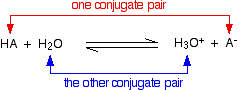# What is the conjugate pair theorem?

Apr 17, 2015In an acid-base neutralization, an acid and a base react to form water and salt.

In order for the reaction to carry out, there must be the transfer of protons between acids and bases. Proton acceptors and proton donors are the basis for these reactions, and are also referred to as conjugate bases and acids.Apr 17, 2015

Although the Chemistry is interesting, the Conjugate Pair Theorem you want in algebra is:

For any polynomial with real coefficients, if $a + b i$ ($b \ne 0$) is a zero of the polynomial, then $a - b i$ is also a zero.

$a - b i$ and $a + b i$ are conjugates of each other, so they form a conjugate pair.

Another way of stating the Conjugate Pair Theorem is:

For any polynomial with real coefficients, imaginary zeros occur in conjugate pairs.

(By definition: a complex number $a + b i$ is imaginary if and only if $b \ne 0$.)